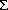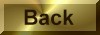Practice Problem 4

Calculate the standard-state entropy of reaction for the following reactions and explain the sign ofS° for each reaction.

(a) Hg(lHg(g)

(b) 2NO2(g)N2O4(g)

(c) N2(g) + O2(g)2NO(g)

Solution

(a) Using a standard-state entropy data table, we find the following information:

Compound            S°(J/mol-K)

Hg(l)                         76.02

Hg(s)                      174.96

The balanced equation states that 1 mole of mercury vapor is produced for each mole of liquid mercury that boils.  The standard-state entropy of reaction is therefore calculated as follows:So =So(products) -So(reactants)

= [1 mol Hg(g) x 174.96 J/mol-K] - [1 mol Hg(l) x 76.02 J/mol-K]

= 98.94 J/K

The sign ofSo is positive because this process transforms a liquid into a gas, which is inherently more disordered.

(b) Using a standard-state entropy data table, we find the following information:

Compound            S°(J/mol-K)

NO2(g)                     240.06

N2O4(g)                     304.29

In this equation, 1 mole of N2O4 is formed for every mole of NO2 consumed, and the value ofSo is calculated as follows:So =So(products) -So(reactants)

= [1 mol N2O4(g) x 304.29 J/mol-K] - [2 mol NO2(l) x 240.06 J/mol-K]

= -175.83 J/K

The sign ofSo is negative because two molecules combine in this reaction to form a larger, more ordered product.

(c) Using a standard-state entropy data table, we find the following information:

Compound            S°(J/mol-K)

NO(g)                        210.76

N2(g)                              191.61

O2(g)                       205.14

The balanced equation for this reaction indicates that 2 mole of NO are produced when 1 mole of N2 reacts with 1 mole of  O2 .   Thus, the standard-state entropy of reaction is calculated as follows:So =So(products) -So(reactants)

= [2 mol NO x 210.76 J/mol-K] - [1 mol N2 x 191/61 J/mol-K + 1 mol O2 x 205.14 J/mol-K]

= 24.77 J/KSo for this reaction is small but positive because the product of the reaction (NO) is slightly more disordered that the reactants ( N2 and  O2).